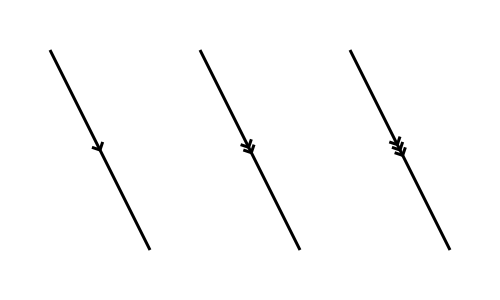# Paratick

By Martin McBride, 2022-01-18
Tags: geometry paratick
Categories: generativepy generative artThe ParallelMarker class is a special Shape that adds a small arros marker to a line, of the type often used in geometric diagrams to show that two lines are parallel. They look like this:You can add 1, 2 or 3 markers.

The TickMarker only draws the tick, it doesn't draw the line itself. This would normally be draw using a Line object, Polygon object, or similar.

## ParallelMarker class

ParallelMarker is derived from the Shape class. It adds several methods:

• of_start_end
• with_length
• with_count
• with_gap

### of_start_end

Creates a marker based on a start and end points.

of_start_end(a, b)

Parameter Type Description
a (number, number) Tuple (x, y) for start of line
b (number, number) Tuple (x, y) for end of line

This will draw an tick marker for the line formed by ab. The marker will be halfway between points a and b, and the arrow will point towards b.

### with_length

Sets the length of the marker.

with_length(length)

Parameter Type Description
length number Length of marker in user units.

The default is 4.

### with_count

Sets the number of arrows in the marker.

with_count(count)

Parameter Type Description
count int Number of arrows

This can be used to draw a single, double, or triple arrows. Permitted values are 1, 2, or 3. Default is 1.

### with_gap

Sets the gap between the arrows if count > 1.

with_gap(gap)

Parameter Type Description
gap number Gap between arrows in user units.

Sets the spacing of the arrows. This is only relevant if there is more than one arrow (ie if count > 1), otherwise it is ignored. The default is 1.

## paratick function

This older paratick function is deprecated as of GenerativePy 3.1.

paratick(ctx, a, b, count=1, length=4, gap=1)

Parameter Type Description
ctx Context The Pycairo Context to draw to
a (number, number) Tuple (x, y) for point a
b (number, number) Tuple (x, y) for point b
count int Number of ticks on the line, 1, 2 or 3.
length number length of the tick in user units.
gap number Gap between ticks in user units.

Draws an tick across the line ab. The tick is half way between points a and b, and points towards b.

## Example

See the example in the angle_marker article.